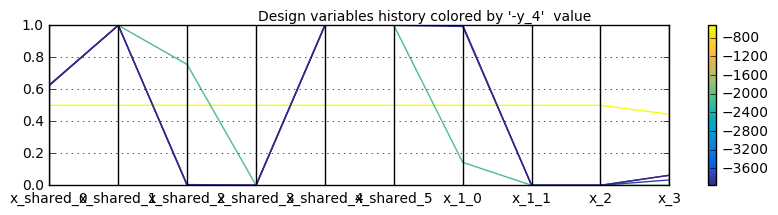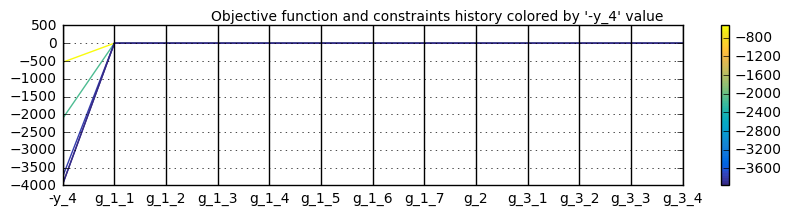# Parallel coordinates¶

## Preliminaries: instantiation and execution of the MDO scenario¶

Let’s start with the following code lines which instantiate and execute the MDOScenario :

from gemseo.api import create_discipline, create_scenario

formulation = 'MDF'

disciplines = create_discipline(["SobieskiPropulsion", "SobieskiAerodynamics",
"SobieskiMission", "SobieskiStructure"])

scenario = create_scenario(disciplines,
formulation=formulation,
objective_name="y_4",
maximize_objective=True,
design_space="design_space.txt")

scenario.set_differentiation_method("user")

algo_options = {'max_iter': 10, 'algo': "SLSQP"}
for constraint in ["g_1","g_2","g_3"]:

scenario.execute(algo_options)


## ParallelCoordinates¶

### Description¶

The ParallelCoordinates post processing builds parallel coordinates plots among design variables, outputs functions and constraints

The ParallelCoordinates portray the design variables history during the scenario execution. Each vertical coordinate is dedicated to a design variable, normalized by its bounds.

A polyline joins all components of a given design vector and is colored by objective function values. This highlights correlations between values of the design variables and values of the objective function.

x- and y- figure sizes can be changed in option. It is possible either to save the plot, to show the plot or both.

### Options¶

• extension, str - file extension

• figsize_x, Unknown - X size of the figure Default value = 10

• figsize_y, Unknown - Y size of the figure Default value = 2

• file_path, str - the base paths of the files to export

• save, bool - if True, exports plot to pdf

• show, bool - if True, displays the plot windows

## Case of the MDF formulation¶

To plot the parallel coordinates, use the API method execute_post() with the keyword "ParallelCoordinates" and additional arguments concerning the type of display (file, screen, both):

scenario.post_process("ParallelCoordinates", save=True, show=False, file_path="mdf" )


Figures Quadratic approximation on the Sobieski use case for the MDF formulation and Quadratic approximation on the Sobieski use case for the MDF formulation show parallel coordinates respectively of the design variables and the constraints, colored by objective function value. The minimum objective value, in dark blue, corresponds to satisfied constraints.Parallel coordinates of design variables on the Sobieski use case for the MDF formulationParallel coordinates of constraints on the Sobieski use case for the MDF formulation